Select Page

Time Value of Money

Time Value of Money states that dollar today is worth more than will tomorrow. Because Inflation (Persistent rise in the general price level over a period of time) and Opportunity Cost (The cost of next best alternative forgone):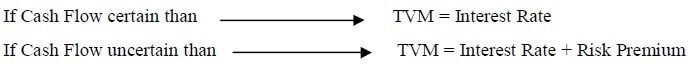The Interest

Fee paid on borrowed money. Compensation to lender for foregoing other useful investment. Equilibrium price at which demand and supply of fund meetI = Interest (The charge for the privilege of borrowing money, typically expressed as an annual percentage rate APR)

RR = Real Rate (An absolutely risk-free investment over a specified period of time)

IF = Inflation Factor (The rate at which the general level of prices for goods and services is rising)

DR = Default Risk (Default risks that companies or individuals will be unable to make the required payments on their debt obligations)

MR = Maturity Risk (Risk associated with interest rate uncertainty. The longer the time to maturity, the higher the premium)

Types of Interest

It is cost to borrow money.  The rent one pays for the use of money is called the interestThere are three types of interest

1. Simple Interest

• Interest calculated on principal amount only
• Although the interest rate is often specified for a year, it may be specified for a week, a month, or a quarter, etc.SIn = Simple Interest for n time period                                                                                             P = Principal Amount

i = Rate of Interest                                                                                                                              n = Time period

Example # 1:

Mr. Salman has three options for investment. Option first offered by Allied Bank is deposit Rs. 100 in a five-year certificate of deposit paying 10% simple interest. How much will have at the end of the five-year?2. Discrete Compound Interest

Interest on principal as well as on interest is called compound interest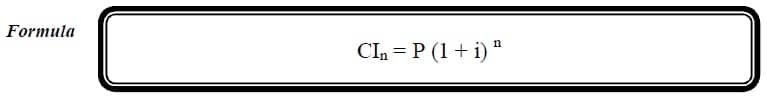CIn = Compound Interest for n time period                                                                                    P = Principal Amount

i = Rate of Interest                                                                                                                              n = Time period

Example # 2:

Mr. Salman has second option offered by National Bank is deposit Rs. 100 in a five-year certificate of deposit paying 10% discrete compound interest. How much will have at the end of the five-year? Find out the difference of interest between compound and simple interest?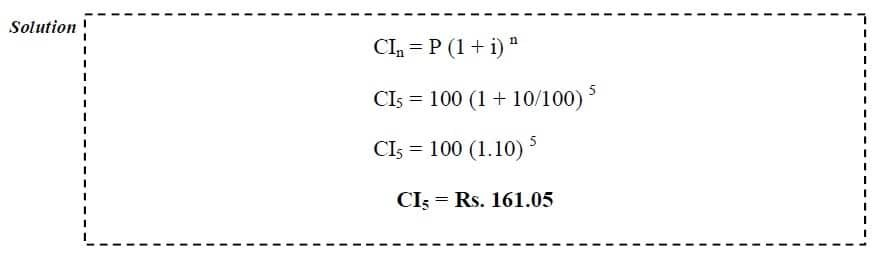Difference of Interest = Compound interest – Simple Interest

Difference of Interest = 161.05 – 150 = Rs. 11.05

This mean interest on principal is Rs. 50 and interest on interest is Rs. 11.05

3. Continuous (Exponential) Compound Interest

• As name suggested that it has exponential increase rate of interest
• When interest is compounded “many times”, we say that the interest is compounded continuously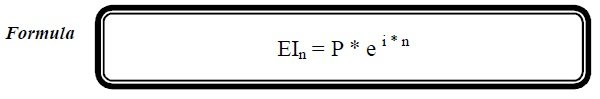Example # 3:

Last option having by Mr. Salman which offered by United Bank is deposit Rs. 100 in a five-year certificate of deposit paying 10% continuous compound interest. How much will have at the end of the five-year?  Which is best choice?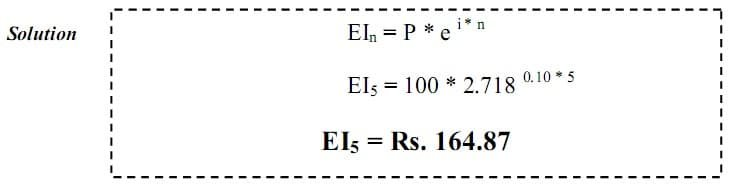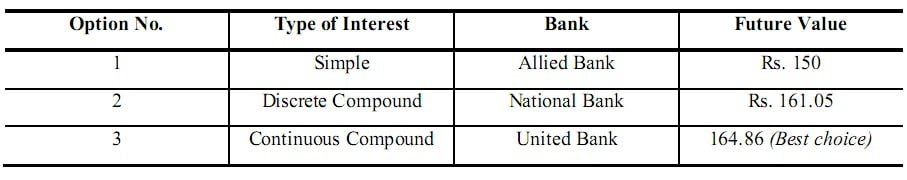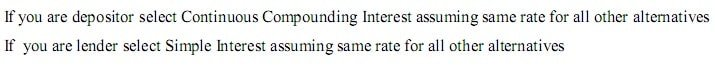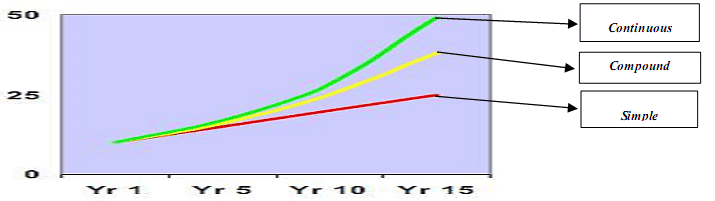>> Practice Time Value of Money MCQs

>>> Practice Time Value of Money MCQs

Types of Interest Rates

There are three types of interest are generally used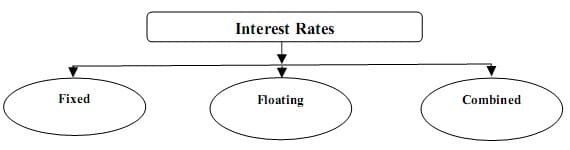1. Fixed Interest Rates

Stated and unchanged rate of interests

2. Floating / Market Interest Rates

Fluctuating rate of interest based on market conditions

3. Combined / Dictated Interest Rates

Mix of fixed and floating rate of interests

Investment Evaluation by Time Value of Money

There are two Time Value of Money techniques are used to evaluate investments>> Practice Time Value of Money Quiz 1 and Quiz 2.